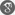##### Cite as:
Kouame Beranger Edja, Koffi N'guessan, Brou Jean-Claude Koua, Kidjegbo Augustin Toure, Numerical Quenching for Heat Equations with Coupled Nonlinear Boundary Flux, Int. J. Anal. Appl., 17 (6) (2019), 1034-1051.

#### Abstract

In this paper, we study a numerical approximation of the following problem ut = uxx, vt = vxx, 0 < x < 1, 0 < t < T; ux(0, t) = u−m(0, t) + v−p(0, t), vx(0, t) = u−q (0, t) + v−n(0, t) and ux(1, t) = vx(1, t) = 0, 0 < t < T, where m, p, q and n are parameters. We prove that the solution of a semidiscrete form of above problem quenches in a finite time only at first node of the mesh. We show that the time derivative of the solution blows up at quenching node. Some conditions under which the non-simultaneous or simultaneous quenching occurs for the solution of the semidiscrete problem are obtained. We establish the convergence of the quenching time. Finally, some numerical results to illustrate our analysis are given.

#### References

1. K.A. Adou, K.A. Tour´e, A. Coulibaly, Numerical study of the blow-up time of positive solutions of semilinear heat equations, Far East J. Appl. Math., 4 (2018), 91-308.2. T. K. Boni, Extinction for discretizations of some semilinear parabolic equations, C. R. Acad. Sci. Paris, 333 (2001), 79-800.3. T. K. Boni, H. Nachid, D. Nabongo, Quenching time of semilinear heat equations, Miskolc Math. Notes, 11 (2010), 27-41.4. C.Y. Chan, S.I. Yuen, Parabolic problems with nonlinear absorptions and releases at the boundaries, Appl. Math. comput., 121 (2001), 203-209.5. K.B. Edja, K.A. Tour´e, B. J.-C. Koua, Numerical Blow-up for a Heat Equation with Nonlinear Boundary Conditions, J. Math. Res., 10 (2018), 119-128.6. R. Ferreira, A. de Pablo, M.P. LLanos, J.D. Rossi, Incomplete quenching in a system of heat equations coupled at the boundary, J. Math. Anal. Appl., 346 (2008), 1145-154.7. R.H. Ji, C.Y. Quc, L.D. Wang, Simultaneous and non-simultaneous quenching for coupled parabolic system, Appl. Anal., 94 (2015), 233-250.8. H. Kawarada, On Solutions of Initial-Boundary Problem for ut = uxx + 1 1−u , Publ. RIMS, Kyoto Univ., 10 (1975), 729-736.9. H.A. Levine, The Quenching of Solutions of Linear Parabolic and Hyperbolic Equations with Nonlinear Boundary Conditions, SIAM J. Math. Anal., 4 (1983), 1139-1153.10. H.A. Levine, J.T. Montgomer The quenching of solutions of some nonlinear parabolic equations, SIAM J. Math. Anal., 11 (1980), 842-847.11. Liang K.W, Lin AP, Tan RCE. Numerical Solution of Quenching Problems Using Mesh-Dependent Variable Temporal Steps, Appl. Numer. Math., 57 (2007), 791-800.12. D. Nabongo, T.K. Boni, Quenching for semidiscretizations of a heat equation with a singular boundary condition, Asymptotic Anal., 59 (2008), 27-38.13. K.C. N’dri, K.A. Tour´e, G. Yoro, Numerical blow-up time for a parabolic equation with nonlinear boundary conditions, Int. J. Numer. Methods Appl., 17 (2018), 141-160.14. H. Pei, Z. Li, Quenching for a parabolic system with general singular terms, J. Nonlinear Sci. Appl., 7 (2016), 1-10.15. B. Seluk, Quenching behavior of a semilinear reaction-diffusion system with singular boundary condition, Turk. J. Math., 40 (2016), 166-180.16. M. Taha, K. Toure, E. Mensah, Numerical approximation of the blow-up time for a semilinear parabolic equation with nonlinear boundary equation, Far East J. Appl. Math., 60 (2012), 125-167.17. S.N. Zheng, X.F. Song, Quenching rates for heat equations with coupled nonlinear boundary flux, Sci. China Ser. A., 51 (2008), 1631-1643.